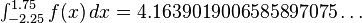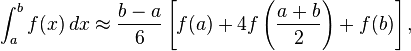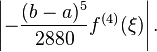The integrals encountered in a basic calculus course are deliberately chosen for simplicity; those found in real applications are not always so accommodating. Some integrals cannot be found exactly, some require special functions which themselves are a challenge to compute, and others are so complex that finding the exact answer is too slow. This motivates the study and application of numerical methods for approximating integrals, which today use floating-point arithmetic on digital electronic computers. Many of the ideas arose much earlier, for hand calculations; but the speed of general-purpose computers like the ENIAC created a need for improvements.

The goals of numerical integration are accuracy, reliability, efficiency, and generality. Sophisticated methods can vastly outperform a naive method by all four measures (Dahlquist & Björck 2008; Kahaner, Moler & Nash 1989; Stoer & Bulirsch 2002). Consider, for example, the integralwhich has the exact answer 9425 = 3.76. (In ordinary practice the answer is not known in advance, so an important task — not explored here — is to decide when an approximation is good enough.) A “calculus book” approach divides the integration range into, say, 16 equal pieces, and computes function values.

 x f(x) x f(x) −2.00 −1.50 −1.00 −0.50 0.00 0.50 1.00 1.50 2.00 2.22800 2.45663 2.67200 2.32475 0.64400 −0.92575 −0.94000 −0.16963 0.83600 −1.75 −1.25 −0.75 −0.25 0.25 0.75 1.25 1.75 2.33041 2.58562 2.62934 1.64019 −0.32444 −1.09159 −0.60387 0.31734Numerical quadrature methods: ■ Rectangle, ■ Trapezoid, ■ Romberg, ■ Gauss

Using the left end of each piece, the rectangle method sums 16 function values and multiplies by the step width, h, here 0.25, to get an approximate value of 3.94325 for the integral. The accuracy is not impressive, but calculus formally uses pieces of infinitesimal width, so initially this may seem little cause for concern. Indeed, repeatedly doubling the number of steps eventually produces an approximation of 3.76001. However, 218 pieces are required, a great computational expense for such little accuracy; and a reach for greater accuracy can force steps so small that arithmetic precision becomes an obstacle.

A better approach replaces the horizontal tops of the rectangles with slanted tops touching the function at the ends of each piece. This trapezium rule is almost as easy to calculate; it sums all 17 function values, but weights the first and last by one half, and again multiplies by the step width. This immediately improves the approximation to 3.76925, which is noticeably more accurate. Furthermore, only 210 pieces are needed to achieve 3.76000, substantially less computation than the rectangle method for comparable accuracy.

Romberg’s method builds on the trapezoid method to great effect. First, the step lengths are halved incrementally, giving trapezoid approximations denoted by T(h0), T(h1), and so on, where hk+1 is half of hk. For each new step size, only half the new function values need to be computed; the others carry over from the previous size (as shown in the table above). But the really powerful idea is to interpolate a polynomial through the approximations, and extrapolate to T(0). With this method a numerically exact answer here requires only four pieces (five function values)! The Lagrange polynomial interpolating {hk,T(hk)}k=0…2 = {(4.00,6.128), (2.00,4.352), (1.00,3.908)} is 3.76+0.148h2, producing the extrapolated value 3.76 at h = 0.

Gaussian quadrature often requires noticeably less work for superior accuracy. In this example, it can compute the function values at just two x positions, ±2√3, then double each value and sum to get the numerically exact answer. The explanation for this dramatic success lies in error analysis, and a little luck. An n-point Gaussian method is exact for polynomials of degree up to 2n−1. The function in this example is a degree 3 polynomial, plus a term that cancels because the chosen endpoints are symmetric around zero. (Cancellation also benefits the Romberg method.)

Shifting the range left a little, so the integral is from −2.25 to 1.75, removes the symmetry. Nevertheless, the trapezoid method is rather slow, the polynomial interpolation method of Romberg is acceptable, and the Gaussian method requires the least work — if the number of points is known in advance. As well, rational interpolation can use the same trapezoid evaluations as the Romberg method to greater effect.

Method Points Rel. Err. Value Trapezoid Romberg Rational Gauss 1048577 257 129 36 −5.3×10−13 −6.3×10−15 8.8×10−15 3.1×10−15In practice, each method must use extra evaluations to ensure an error bound on an unknown function; this tends to offset some of the advantage of the pure Gaussian method, and motivates the popular Gauss–Kronrod quadrature formulae. Symmetry can still be exploited by splitting this integral into two ranges, from −2.25 to −1.75 (no symmetry), and from −1.75 to 1.75 (symmetry). More broadly, adaptive quadrature partitions a range into pieces based on function properties, so that data points are concentrated where they are needed most.

Simpson’s rule, named for Thomas Simpson (1710–1761), uses a parabolic curve to approximate integrals. In many cases, it is more accurate than the trapezoidal rule and others. The rule states thatwith an error ofThe computation of higher-dimensional integrals (for example, volume calculations) makes important use of such alternatives as Monte Carlo integration.

A calculus text is no substitute for numerical analysis, but the reverse is also true. Even the best adaptive numerical code sometimes requires a user to help with the more demanding integrals. For example, improper integrals may require a change of variable or methods that can avoid infinite function values, and known properties like symmetry and periodicity may provide critical leverage.

RELATED POST ::1.Belajar Komputer